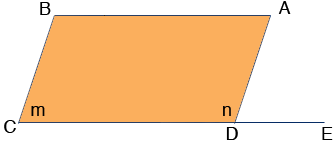SEARCH HOMEMath Central Quandaries & QueriesQuestion from amber, a student: i cant figure this out: the measure of one interior angle of a parallelogram is 42 degrees more than twice the measure of another angle. Find the measure of each angle. if u can help, thanx!Hi Amber,

In the parallelogram ABCD extend the side CD to E. Let m be the measure of the angle BCD and n be the measure of the angle CDA.Since BC and DA are parallel and CE is a transversal the measure of the angle ADE is also m. Thus

m + n = 180 degrees.

Now, what else do you know?

the measure of one interior angle of a parallelogram is 42 degrees more than twice the measure of another angle

In my diagram n is larger than m and the line above says that n is more than 2m. In fact it is 42 degrees more than 2m so

n = 2m + 42

Can you now solve these equations for m and n?

PennyMath Central is supported by the University of Regina and The Pacific Institute for the Mathematical Sciences.